# MAFS.8.NS.1.2Archived StandardExport Print
Use rational approximations of irrational numbers to compare the size of irrational numbers, locate them approximately on a number line diagram, and estimate the value of expressions (e.g., π²). For example, by truncating the decimal expansion of √2, show that √2 is between 1 and 2, then between 1.4 and 1.5, and explain how to continue on to get better approximations.
General Information
Subject Area: Mathematics
Domain-Subdomain: The Number System
Cluster: Level 2: Basic Application of Skills & Concepts
Cluster: Know that there are numbers that are not rational, and approximate them by rational numbers. (Supporting Cluster) -

Clusters should not be sorted from Major to Supporting and then taught in that order. To do so would strip the coherence of the mathematical ideas and miss the opportunity to enhance the major work of the grade with the supporting clusters.

Date of Last Rating: 02/14
Status: State Board Approved - Archived
Assessed: Yes
Test Item Specifications

• Assessment Limits :
All irrational numbers may be used, excluding e. Irrational expressions should only use one operation.
• Calculator :

No

• Context :

No context

Sample Test Items (4)
• Test Item #: Sample Item 1
• Question:

What is the approximate value of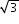, to the nearest whole number?

• Difficulty: N/A
• Type: EE: Equation Editor

• Test Item #: Sample Item 2
• Question:

What is the approximate value of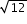?

• Difficulty: N/A
• Type: MC: Multiple Choice

• Test Item #: Sample Item 3
• Question:

A number line is shown.

Place the following numbers in the proper location on the number line.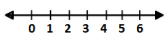••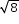•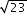• Difficulty: N/A
• Type: GRID: Graphic Response Item Display

## Related Courses

This benchmark is part of these courses.
1205050: M/J Accelerated Mathematics Grade 7 (Specifically in versions: 2014 - 2015, 2015 - 2020, 2020 - 2022, 2022 and beyond (current))
1205070: M/J Grade 8 Pre-Algebra (Specifically in versions: 2014 - 2015, 2015 - 2022, 2022 and beyond (current))
1204000: M/J Foundational Skills in Mathematics 6-8 (Specifically in versions: 2014 - 2015, 2015 - 2022, 2022 and beyond (current))
1200410: Mathematics for College Success (Specifically in versions: 2014 - 2015, 2015 - 2022 (course terminated))
1200700: Mathematics for College Algebra (Specifically in versions: 2014 - 2015, 2015 - 2022 (course terminated))
7812030: Access M/J Grade 8 Pre-Algebra (Specifically in versions: 2014 - 2015, 2015 - 2018, 2018 - 2019, 2019 - 2022, 2022 and beyond (current))

## Related Access Points

Alternate version of this benchmark for students with significant cognitive disabilities.

## Related Resources

Vetted resources educators can use to teach the concepts and skills in this benchmark.

## Formative Assessments

Repeating Decimals:

This lesson unit is intended to help you assess how well students are able to:

• Translate between decimal and fraction notation, particularly when the decimals are repeating.
• Create and solve simple linear equations to find the fractional equivalent of a repeating decimal.
• Understand the effect of multiplying a decimal by a power of 10.

Type: Formative Assessment

The Irrational Beauty of the Golden Ratio:

Students are asked to find and interpret lower and upper bounds of an irrational expression using a calculator.

Type: Formative Assessment

Approximating Irrational Numbers:

Students are asked to plot the square root of eight on three number lines, scaled to progressively more precision.

Type: Formative Assessment

Locating Irrational Numbers:

Students are asked to graph three different irrational numbers on number lines.

Type: Formative Assessment

Comparing Irrational Numbers:

Students are asked to estimate the value of several irrational numbers using a calculator and order them on a number line.

Type: Formative Assessment

## Lesson Plans

Testing Imperfection:

Students will use number lines to approximate square roots of non-perfect squares.

Type: Lesson Plan

Repeating Decimals:

This lesson unit is intended to help you assess how well students are able to translate between decimal and fraction notation, particularly when the decimals are repeating, create and solve simple linear equations to find the fractional equivalent of a repeating decimal, and understand the effect of multiplying a decimal by a power of 10.

Type: Lesson Plan

Pin the Irrational "Tail" on the Number Line:

Students will use their knowledge of perfect squares and square roots to determine a rational number to approximate an irrational number and find their locations on a number line. They will complete an activity which guides them to zoom further into a number line to find more accurate approximations for irrational numbers. They will conclude that between two rational numbers is another rational number and therefore the further the place value in our approximation, the more accurate its location on the number line will be.

Type: Lesson Plan

Non-perfect square roots as rational numbers:

Understanding Irrational numbers that can be written as rational numbers can assist students and their understanding of the real number system. Irrational numbers can be approximated as rational numbers but not written as rational numbers. This lesson provides practice in these ideas.

Type: Lesson Plan

It's Hip to Be (an Imperfect) Square!:

This lesson allows students to explore and estimate the values of imperfect squares, using perfect square anchors and number lines as resources. The conversations throughout the lesson will also emphasize that imperfect squares are irrational numbers that must be estimated to compare.

Type: Lesson Plan

Irrational Numbers on the Number Line:

When students plot irrational numbers on the number line, it helps reinforce the idea that they fit into a number system that includes the more familiar integer and rational numbers. This is a good time for teachers to start using the term "real number line" to emphasize the fact that the number system represented by the number line is the real numbers. When students begin to study complex numbers in high school, they will encounter numbers that are not on the real number line (and are, in fact, on a "number plane"). This task could be used for assessment, or if elaborated a bit, could be used in an instructional setting.

Comparing Rational and Irrational Numbers:

Students are given a pair of numbers. They are asked to determine which is larger, and then justify their answer. The numbers involved are rational numbers and common irrational numbers, such p and square roots. This task can be used to either build or assess initial understandings related to rational approximations of irrational numbers.

## Student Center Activity

Students can practice answering mathematics questions on a variety of topics. With an account, students can save their work and send it to their teacher when complete.

Type: Student Center Activity

## Tutorials

Comparing Irrational Numbers With and Without a Calculator:

In this video, you will practice comparing an irrational number to a percent. First you will try it without a calculator. Then you will check your answer using a calculator.

Type: Tutorial

Approximating Square Roots to the Hundredths:

In this video, you will learn how to approximate a square root to the hundredths place.

Type: Tutorial

How to Approximate Square Roots:

In this video, you will practice approximating square roots of numbers that are not perfect squares. You will find the perfect square below and above to approximate the value of the square root between two whole numbers.

Type: Tutorial

## MFAS Formative Assessments

Approximating Irrational Numbers:

Students are asked to plot the square root of eight on three number lines, scaled to progressively more precision.

Comparing Irrational Numbers:

Students are asked to estimate the value of several irrational numbers using a calculator and order them on a number line.

Locating Irrational Numbers:

Students are asked to graph three different irrational numbers on number lines.

The Irrational Beauty of the Golden Ratio:

Students are asked to find and interpret lower and upper bounds of an irrational expression using a calculator.

## Student Resources

Vetted resources students can use to learn the concepts and skills in this benchmark.

Irrational Numbers on the Number Line:

When students plot irrational numbers on the number line, it helps reinforce the idea that they fit into a number system that includes the more familiar integer and rational numbers. This is a good time for teachers to start using the term "real number line" to emphasize the fact that the number system represented by the number line is the real numbers. When students begin to study complex numbers in high school, they will encounter numbers that are not on the real number line (and are, in fact, on a "number plane"). This task could be used for assessment, or if elaborated a bit, could be used in an instructional setting.

Comparing Rational and Irrational Numbers:

Students are given a pair of numbers. They are asked to determine which is larger, and then justify their answer. The numbers involved are rational numbers and common irrational numbers, such p and square roots. This task can be used to either build or assess initial understandings related to rational approximations of irrational numbers.

## Student Center Activity

Students can practice answering mathematics questions on a variety of topics. With an account, students can save their work and send it to their teacher when complete.

Type: Student Center Activity

## Tutorials

Comparing Irrational Numbers With and Without a Calculator:

In this video, you will practice comparing an irrational number to a percent. First you will try it without a calculator. Then you will check your answer using a calculator.

Type: Tutorial

Approximating Square Roots to the Hundredths:

In this video, you will learn how to approximate a square root to the hundredths place.

Type: Tutorial

How to Approximate Square Roots:

In this video, you will practice approximating square roots of numbers that are not perfect squares. You will find the perfect square below and above to approximate the value of the square root between two whole numbers.

Type: Tutorial

## Parent Resources

Vetted resources caregivers can use to help students learn the concepts and skills in this benchmark.